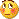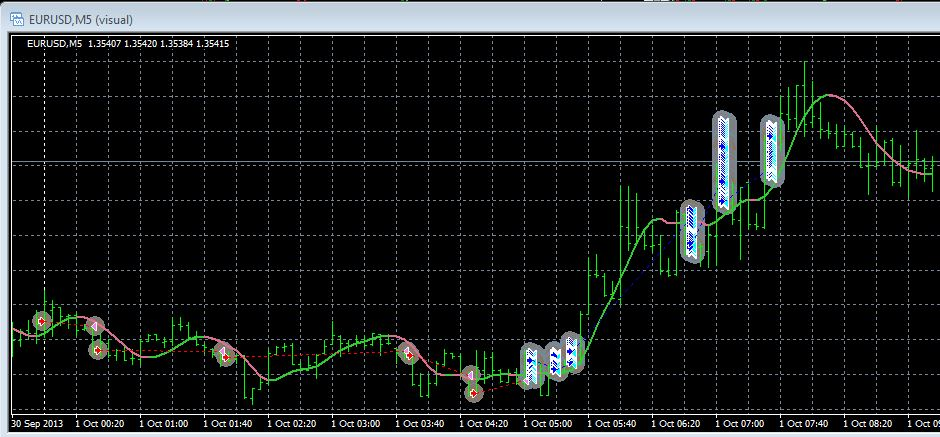Coding help - page 39092

Hello MT4 coders,

I wonder if someone could help me. I am trying to implement "Hull moving average 2 strict nmc"indicator into an EA.

The problem what I am facing is that the Indicator is loaded over and over into MT4 according to the report log, further more the EA is not opening a trade. As far as I know a problem with the iCustom indicator call may be the root cause.

This is what I try to do:

The EA should go LONG when Hull moving average 2 strict nmc is green and short when Hull moving average 2 strict nmc is red.

extern int HMAPeriod = 35;

extern int HMAPrice = 0; // PRICE_CLOSE

extern double HMASpeed = 2;

[/CODE]

[CODE]

// Forex TSD Hull Moving Average

double hma0 = iCustom(Symbol(), 0, "Hull moving average 2 strict nmc", HMAPeriod, HMAPrice, HMASpeed, 0, 2, 0);

double hma1 = iCustom(Symbol(), 0, "Hull moving average 2 strict nmc", HMAPeriod, HMAPrice, HMASpeed, 0, 2, 1);

double hma2 = iCustom(Symbol(), 0, "Hull moving average 2 strict nmc", HMAPeriod, HMAPrice, HMASpeed, 0, 2, 2);

Buy = (hma0 > hma1 && hma1 > hma2);

Sell = (hma0 < hma1 && hma1 < hma2);246209

tfi_markets:
Hello MT4 coders,

I wonder if someone could help me. I am trying to implement "Hull moving average 2 strict nmc"indicator into an EA.

The problem what I am facing is that the Indicator is loaded over and over into MT4 according to the report log, further more the EA is not opening a trade. As far as I know a problem with the iCustom indicator call may be the root cause.

This is what I try to do:

The EA should go LONG when Hull moving average 2 strict nmc is green and short when Hull moving average 2 strict nmc is red.

extern int HMAPeriod = 35;

extern int HMAPrice = 0; // PRICE_CLOSE

extern double HMASpeed = 2;

[/CODE]

[CODE]

// Forex TSD Hull Moving Average

double hma0 = iCustom(Symbol(), 0, "Hull moving average 2 strict nmc", HMAPeriod, HMAPrice, HMASpeed, 0, 2, 0);

double hma1 = iCustom(Symbol(), 0, "Hull moving average 2 strict nmc", HMAPeriod, HMAPrice, HMASpeed, 0, 2, 1);

double hma2 = iCustom(Symbol(), 0, "Hull moving average 2 strict nmc", HMAPeriod, HMAPrice, HMASpeed, 0, 2, 2);

Buy = (hma0 > hma1 && hma1 > hma2);

Sell = (hma0 < hma1 && hma1 < hma2);

tfi_markets

You are missing one parameter (the first one, the TimeFrame parameter). Change the iCustom() call to this :

iCustom(Symbol(),0,"Hull moving average 2 strict nmc", "", HMAPeriod, HMAPrice,HMASpeed,0,2,0);

and it will work OK92

thank you very much for your help!

I have implemented your suggestion, the error is gone, which is very good. But the EA is unfortunately still not open a trade. I would appreciate if you could review the order logic, maybe I am doing something wrong here?

int Extra_Pips=1;

extern int HMA_Period=21;

extern int HMA_Price=PRICE_CLOSE; //0

extern double HMA_Speed= 2;

//+------------------------------------------------------------------+

//| ORDER Logic / Indicators |

//+------------------------------------------------------------------+

if(openedOrders<=0)

{

// Forex TSD Hull Moving Average

double hma0 = iCustom(Symbol(),0,"Hull moving average 2 strict nmc", "", HMA_Period, HMA_Price, HMA_Speed,0,2,0);

double hma1 = iCustom(Symbol(),0,"Hull moving average 2 strict nmc", "", HMA_Period, HMA_Price, HMA_Speed,0,2,1);

double hma2 = iCustom(Symbol(),0,"Hull moving average 2 strict nmc", "", HMA_Period, HMA_Price, HMA_Speed,0,2,2);

//+------------------------------------------------------------------+

//+------------------------------------------------------------------+

if(hma0>hma1 && hma1>hma2)

{

// Wait some pips

double pipsExtra1=Extra_Pips*Point; // Get distance from cross signal

return(0);

}

//+------------------------------------------------------------------+

//| SELL |

//+------------------------------------------------------------------+

if(hma0<hma1 && hma1<hma2)

{

// Wait some pips

double pipsExtra2=Extra_Pips*Point; // Get distance from cross signal

OpenSell();

return(0);

}

}161

Would someone please help me with the code for calculating lot size for different pairs such that each pip profit is 10 units of currency? For example if "ProfitPerPip = 10" then the lot for EURUSD would be 1.00

Thank you.246209

tfi_markets:

thank you very much for your help!

I have implemented your suggestion, the error is gone, which is very good. But the EA is unfortunately still not open a trade. I would appreciate if you could review the order logic, maybe I am doing something wrong here?

int Extra_Pips=1;

extern int HMA_Period=21;

extern int HMA_Price=PRICE_CLOSE; //0

extern double HMA_Speed= 2;

//+------------------------------------------------------------------+

//| ORDER Logic / Indicators |

//+------------------------------------------------------------------+

if(openedOrders<=0)

{

// Forex TSD Hull Moving Average

double hma0 = iCustom(Symbol(),0,"Hull moving average 2 strict nmc", "", HMA_Period, HMA_Price,HMA_Speed,0,2,0);

double hma1 = iCustom(Symbol(),0,"Hull moving average 2 strict nmc", "", HMA_Period, HMA_Price, HMA_Speed,0,2,1);

double hma2 = iCustom(Symbol(),0,"Hull moving average 2 strict nmc", "", HMA_Period, HMA_Price, HMA_Speed,0,2,2);

//+------------------------------------------------------------------+

//+------------------------------------------------------------------+

if(hma0>hma1 && hma1>hma2)

{

// Wait some pips

double pipsExtra1=Extra_Pips*Point; // Get distance from cross signal

return(0);

}

//+------------------------------------------------------------------+

//| SELL |

//+------------------------------------------------------------------+

if(hma0<hma1 && hma1<hma2)

{

// Wait some pips

double pipsExtra2=Extra_Pips*Point; // Get distance from cross signal

OpenSell();

return(0);

}

}

tfi_markets

The simplest way is to check trend buffer (buffer 3)

Something like this :

double trendc = iCustom(Symbol(),0,"Hull moving average 2 strict nmc", "", HMA_Period, HMA_Price, HMA_Speed,0,3,1);

double trendp = iCustom(Symbol(),0,"Hull moving average 2 strict nmc", "", HMA_Period, HMA_Price, HMA_Speed,0,3,2);

if (trendc!=trendp)

{

if (trendc == 1) // code for buy

if (trendc == -1) // code for sell

}92

tfi_markets

The simplest way is to check trend buffer (buffer 3)

Something like this :

double trendc = iCustom(Symbol(),0,"Hull moving average 2 strict nmc", "", HMA_Period, HMA_Price, HMA_Speed,0,3,1);

double trendp = iCustom(Symbol(),0,"Hull moving average 2 strict nmc", "", HMA_Period, HMA_Price, HMA_Speed,0,3,2);

if (trendc!=trendp)

{

if (trendc == 1) // code for buy

if (trendc == -1) // code for sell

}

sorry I have to bother you again, thank you very much for your suggestions until now

I have implemented it as follows, but unfortunately it seems not to catch the trend change properly,

and it is running wild in opening buy orders, please see attached screenshot.Code for opening orders:

if(openedOrders<=0)

{

// Forex TSD Hull Moving Average

double trendc = iCustom(Symbol(),0,"Hull moving average 2 strict nmc", "", HMA_Period, HMA_Price, HMA_Speed,0,3,1);

double trendp = iCustom(Symbol(),0,"Hull moving average 2 strict nmc", "", HMA_Period, HMA_Price, HMA_Speed,0,3,2);

if(trendc!=trendp)

{

return(0);

}

if(trendc==-1) // code for sell

{

OpenSell();

return(0);

}

}

//+------------------------------------------------------------------+

//+------------------------------------------------------------------+

{

double lbStop = 0; if(lStopLoss>0) lbStop = NormalizeDouble(MarketInfo(s_symbol,MODE_ASK)-lStopLoss *pPoint*pipMultiplier,digit);

double lbTake = 0; if(lTakeProfit>0) lbTake = NormalizeDouble(MarketInfo(s_symbol,MODE_ASK)+lTakeProfit*pPoint*pipMultiplier,digit);

if(AccountFreeMargin()<(100*Lots)) { Print("We have no money. Free Margin = ",AccountFreeMargin()); return; }

//

//

//

if(!EcnBroker)

else

{

{

int ErrorCode = GetLastError();

string ErrDesc = ErrorDescription(ErrorCode);

Print(ErrLog);

}

}

}

//+------------------------------------------------------------------+

//| Open Sell |

//+------------------------------------------------------------------+

void OpenSell()

{

double lsStop = 0; if(sStopLoss>0) lsStop = NormalizeDouble(MarketInfo(s_symbol,MODE_BID)+sStopLoss *pPoint*pipMultiplier,digit);

double lsTake = 0; if(sTakeProfit>0) lsTake = NormalizeDouble(MarketInfo(s_symbol,MODE_BID)-sTakeProfit*pPoint*pipMultiplier,digit);

if(AccountFreeMargin()<(100*Lots)) { Print("We have no money. Free Margin = ",AccountFreeMargin()); return; }

//+------------------------------------------------------------------+

//| ECN Broker |

//+------------------------------------------------------------------+

if(!EcnBroker)

dummyResult=OrderSend(s_symbol,OP_SELL,LotsOptimized(),MarketInfo(s_symbol,MODE_BID),Slippage*pipMultiplier,lsStop,lsTake,ExpertName,MAGIC,0,clOpenSell);

else

{

int sellTicket = OrderSend(s_symbol,OP_SELL,LotsOptimized(),MarketInfo(s_symbol,MODE_BID),Slippage*pipMultiplier,0,0,ExpertName,MAGIC,0,clOpenSell);

if(sellTicket >= 0)

bool sellOrderMod=OrderModify(sellTicket,OrderOpenPrice(),lsStop,lsTake,0,CLR_NONE);

if(sellOrderMod==false)

{

int ErrorCode = GetLastError();

string ErrDesc = ErrorDescription(ErrorCode);

string ErrAlert=StringConcatenate("Modify Sell Order - Error ",ErrorCode,": ",ErrDesc);

Print(ErrLog);

}

}

}

[/CODE]

Code for closing orders:

[CODE]

void CheckForClose()

{

RefreshRates();

double trendc_c = iCustom(Symbol(),0,"Hull moving average 2 strict nmc", "", HMA_Period, HMA_Price, HMA_Speed,0,3,1);

double trendp_c = iCustom(Symbol(),0,"Hull moving average 2 strict nmc", "", HMA_Period, HMA_Price, HMA_Speed,0,3,2);

for(int i=0;i<OrdersTotal(); i++)

{

if(OrderMagicNumber()!= MAGIC) continue;

if(OrderSymbol() != s_symbol) continue;

if(trendc_c!=trendp_c) // Check Trend

{

{

{

{

int ErrorCode = GetLastError();

string ErrDesc = ErrorDescription(ErrorCode);

string ErrLog=StringConcatenate("Bid: ",MarketInfo(s_symbol,MODE_BID)," Lots: ",OrderLots()," Ticket: ",OrderTicket());

Print(ErrLog);

}

}

break;

}

}

if(trendc_c!=trendp_c) // Check trend

{

if(OrderType()==OP_SELL)

{

if(trendc_c==-1) // is SELL?

{

if(sellClose == false)

{

ErrorCode = GetLastError();

ErrDesc = ErrorDescription(ErrorCode);

ErrAlert=StringConcatenate("Close Sell Order - Error ",ErrorCode,": ",ErrDesc);

Print(ErrLog);

}

}

break;

}

}

}

}Files:
hma-error.jpg  108 kb246209

tfi_markets:

sorry I have to bother you again, thank you very much for your suggestions until now

I have implemented it as follows, but unfortunately it seems not to catch the trend change properly,

and it is running wild in opening buy orders, please see attached screenshot.Code for opening orders:

if(openedOrders<=0)

{

// Forex TSD Hull Moving Average

double trendc = iCustom(Symbol(),0,"Hull moving average 2 strict nmc", "", HMA_Period, HMA_Price, HMA_Speed,0,3,1);

double trendp = iCustom(Symbol(),0,"Hull moving average 2 strict nmc", "", HMA_Period, HMA_Price, HMA_Speed,0,3,2);

if(trendc!=trendp)

{

return(0);

}

if(trendc==-1) // code for sell

{

OpenSell();

return(0);

}

}

//+------------------------------------------------------------------+

//+------------------------------------------------------------------+

{

double lbStop = 0; if(lStopLoss>0) lbStop = NormalizeDouble(MarketInfo(s_symbol,MODE_ASK)-lStopLoss *pPoint*pipMultiplier,digit);

double lbTake = 0; if(lTakeProfit>0) lbTake = NormalizeDouble(MarketInfo(s_symbol,MODE_ASK)+lTakeProfit*pPoint*pipMultiplier,digit);

if(AccountFreeMargin()<(100*Lots)) { Print("We have no money. Free Margin = ",AccountFreeMargin()); return; }

//

//

//

if(!EcnBroker)

else

{

{

int ErrorCode = GetLastError();

string ErrDesc = ErrorDescription(ErrorCode);

Print(ErrLog);

}

}

}

//+------------------------------------------------------------------+

//| Open Sell |

//+------------------------------------------------------------------+

void OpenSell()

{

double lsStop = 0; if(sStopLoss>0) lsStop = NormalizeDouble(MarketInfo(s_symbol,MODE_BID)+sStopLoss *pPoint*pipMultiplier,digit);

double lsTake = 0; if(sTakeProfit>0) lsTake = NormalizeDouble(MarketInfo(s_symbol,MODE_BID)-sTakeProfit*pPoint*pipMultiplier,digit);

if(AccountFreeMargin()<(100*Lots)) { Print("We have no money. Free Margin = ",AccountFreeMargin()); return; }

//+------------------------------------------------------------------+

//| ECN Broker |

//+------------------------------------------------------------------+

if(!EcnBroker)

dummyResult=OrderSend(s_symbol,OP_SELL,LotsOptimized(),MarketInfo(s_symbol,MODE_BID),Slippage*pipMultiplier,lsStop,lsTake,ExpertName,MAGIC,0,clOpenSell);

else

{

int sellTicket = OrderSend(s_symbol,OP_SELL,LotsOptimized(),MarketInfo(s_symbol,MODE_BID),Slippage*pipMultiplier,0,0,ExpertName,MAGIC,0,clOpenSell);

if(sellTicket >= 0)

bool sellOrderMod=OrderModify(sellTicket,OrderOpenPrice(),lsStop,lsTake,0,CLR_NONE);

if(sellOrderMod==false)

{

int ErrorCode = GetLastError();

string ErrDesc = ErrorDescription(ErrorCode);

string ErrAlert=StringConcatenate("Modify Sell Order - Error ",ErrorCode,": ",ErrDesc);

Print(ErrLog);

}

}

}

[/CODE]

Code for closing orders:

[CODE]

void CheckForClose()

{

RefreshRates();

double trendc_c = iCustom(Symbol(),0,"Hull moving average 2 strict nmc", "", HMA_Period, HMA_Price, HMA_Speed,0,3,1);

double trendp_c = iCustom(Symbol(),0,"Hull moving average 2 strict nmc", "", HMA_Period, HMA_Price, HMA_Speed,0,3,2);

for(int i=0;i<OrdersTotal(); i++)

{

if(OrderMagicNumber()!= MAGIC) continue;

if(OrderSymbol() != s_symbol) continue;

if(trendc_c!=trendp_c) // Check Trend

{

{

{

{

int ErrorCode = GetLastError();

string ErrDesc = ErrorDescription(ErrorCode);

string ErrLog=StringConcatenate("Bid: ",MarketInfo(s_symbol,MODE_BID)," Lots: ",OrderLots()," Ticket: ",OrderTicket());

Print(ErrLog);

}

}

break;

}

}

if(trendc_c!=trendp_c) // Check trend

{

if(OrderType()==OP_SELL)

{

if(trendc_c==-1) // is SELL?

{

if(sellClose == false)

{

ErrorCode = GetLastError();

ErrDesc = ErrorDescription(ErrorCode);

ErrAlert=StringConcatenate("Close Sell Order - Error ",ErrorCode,": ",ErrDesc);

Print(ErrLog);

}

}

break;

}

}

}

}A question : why are you closing buy orders when the signal for buy comes in and sell orders when the signal for sell comes in? Shouldn't that be inverted? And I think that it will prevent some things happening now373

Simple question about Mql5, how can we hide the indicator values/labels on top of the subwindow ? In mql4, I know it's with "SetIndexLabel(0,NULL);", but I can't find it for mt5. Thanks a lot.246209

airquest:
Simple question about Mql5, how can we hide the indicator values/labels on top of the subwindow ? In mql4, I know it's with "SetIndexLabel(0,NULL);", but I can't find it for mt5. Thanks a lot.

airquest

Use : PlotIndexSetInteger(0,PLOT_SHOW_DATA,false); for that242

Hi Mladen. I have attached an expert that I used to use before the latest issue of Metatrader. Now it doesn't work.

Any chance you could weave your magic wand over it, & get it working again. If you can I'm happy for anyone to use it....it's a good one (Or was!)

Files: